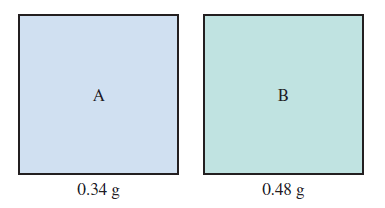# Problem: Consider two gases, A and B, each in a 1.0-L container with both gases at the same temperature and pressure. The mass of gas A in the container is 0.34 g and the mass of gas B in the container is 0.48 g.a. Which gas sample has the most molecules present? Explain.

###### FREE Expert Solution

We’re given 0.34 g of gas A and 0.48 g of gas B, each in a 1.0-L container at the same pressure and volume. We’re being asked which gas sample has the most number of molecules present.

Recall that the ideal gas law is:91% (358 ratings)###### Problem Details

Consider two gases, A and B, each in a 1.0-L container with both gases at the same temperature and pressure. The mass of gas A in the container is 0.34 g and the mass of gas B in the container is 0.48 g.a. Which gas sample has the most molecules present? Explain.